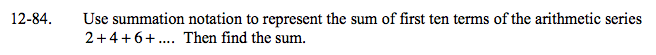### Home > A2C > Chapter 12 > Lesson 12.2.1 > Problem12-84

12-84.

Use summation notation to represent the sum of first ten terms of the arithmetic series 2 + 4 + 6 + …. Then find the sum. Homework Help ✎$\sum_{n=1}^{10}(2n)$